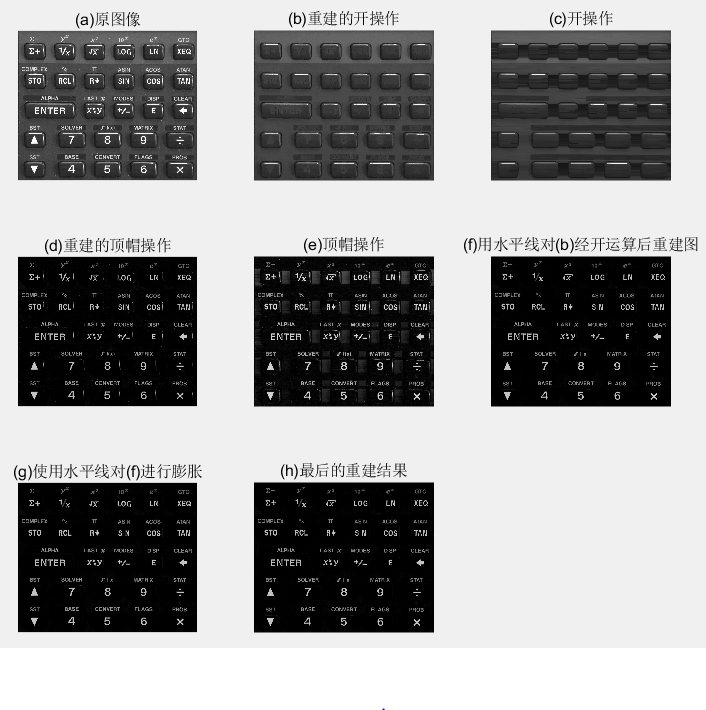# 图像处理 形态学运算

## 数字图像处理：10.形态学运算

2013-08-01 10:33:41 ichangjian 阅读数 3306
• ###### MATLAB图像处理

介绍MATLAB提供的图像处理功能，包括图像基础知识、图像合成、空间变换、邻域和块处理、局部滤波、正交变换、数学形态学、图像分析、图像增强、图像恢复、图像分割、图像配准和图像三维重建等内容。

47课时 342分钟 216人学习 苏金明
免费试看

1．引言2. 基本概念

（1）包含、击中、不击中（2）平移、对称集（3） 腐蚀（4）膨胀（图片来源：艾海舟，王栓，何克忠，基于差分图象的人脸检测，中国图象图形学报，1998; 3(12): 987-992.）

3. 开运算和闭运算

（1）开运算（2）闭运算4. 击中击不中变换HMT(Hit-Miss Transform)（2）4.1细化和粗化

（1）细化（Thin）（2）粗化(Thick)5. 边界和骨架（Boundary and Skeleton）Binary Sobel EdgeDilationErosionOpenCloseThinning by Structure Elemnets of T and L shapes

Form

## 数字图像处理-数学形态学运算(腐蚀与膨胀)

2020-04-08 11:00:20 qq_43055030 阅读数 177
• ###### MATLAB图像处理

介绍MATLAB提供的图像处理功能，包括图像基础知识、图像合成、空间变换、邻域和块处理、局部滤波、正交变换、数学形态学、图像分析、图像增强、图像恢复、图像分割、图像配准和图像三维重建等内容。

47课时 342分钟 216人学习 苏金明
免费试看

# 腐蚀与膨胀

• 腐蚀运算的原理是对数据集中的每个元素在自定义的结构元素(窗口)内寻求最小值来替代中心元素的值。腐蚀运算的物理意义是具有腐蚀数据集合边缘的功能并迫使集合向内收缩，如果窗口足够的大，就能移除那些面积较小、作用不大的物体。
• 膨胀运算与腐蚀运算的原理相反，它是对数据集合中的每个元素在自定义的结构元素内寻找最大值来代替中心元素的值。膨胀运算的物理意义为扩展数据集合的大小并使其向集合外膨胀，适宜的窗口大小可以填充集合内部的孔洞，使集合更加完整。

## 1.数学定义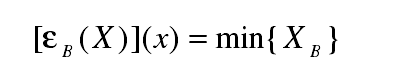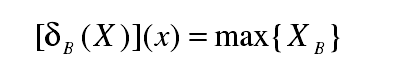## 2. 代码

• 腐蚀代码
//使用 3*3窗口进行滤波
//这里使用了Eigen的矩阵元素定义了结构lgrid
void basicfuc::erosion(lgrid &src, lgrid &dst)
{

int x = src.getX();
int y = src.getY();
for (size_t i = 1; i < x - 1; i++)
{
for (size_t j = 1; j < y - 1; j++)
{
float a;
//top
a = src.getGrid(i - 1, j - 1);
a = src.getGrid(i - 1, j);
a = src.getGrid(i - 1, j + 1);

//middle
a = src.getGrid(i, j - 1);
a = src.getGrid(i, j);
a = src.getGrid(i, j + 1);

//bottom
a = src.getGrid(i + 1, j - 1);
a = src.getGrid(i + 1, j);
a = src.getGrid(i + 1, j + 1);

float minValue = *std::min_element(a, a + 9);
dst.setGrid(i, j, minValue);
}
}
}

• 膨胀代码
//同样采用3*3算子
void basicfuc::dilation(lgrid &src, lgrid &dst )
{

int x = src.getX();
int y = src.getY();
for (size_t i = 1; i < x - 1; i++)
{
for (size_t j = 1; j < y - 1; j++)
{
float a;
//top
a = src.getGrid(i - 1, j - 1);
a = src.getGrid(i - 1, j);
a = src.getGrid(i - 1, j + 1);

//middle
a = src.getGrid(i, j - 1);
a = src.getGrid(i, j);
a = src.getGrid(i, j + 1);

//bottom
a = src.getGrid(i + 1, j - 1);
a = src.getGrid(i + 1, j);
a = src.getGrid(i + 1, j + 1);

float maxValue = *std::max_element(a, a + 9);
dst.setGrid(i, j, maxValue);
}
}
}


## 3.效果

1. 原始图像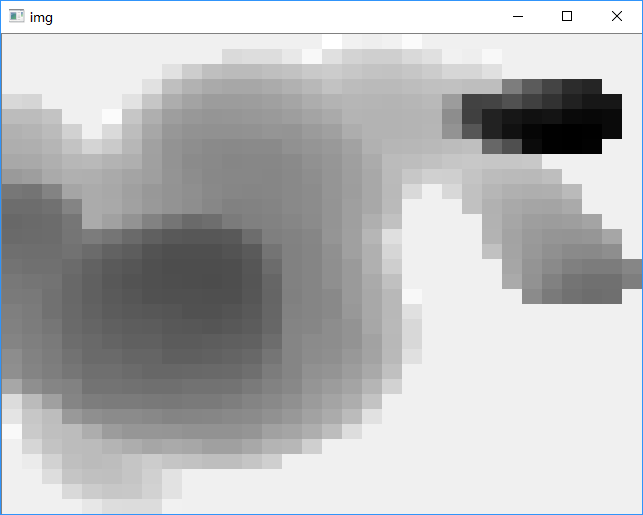1. 腐蚀图像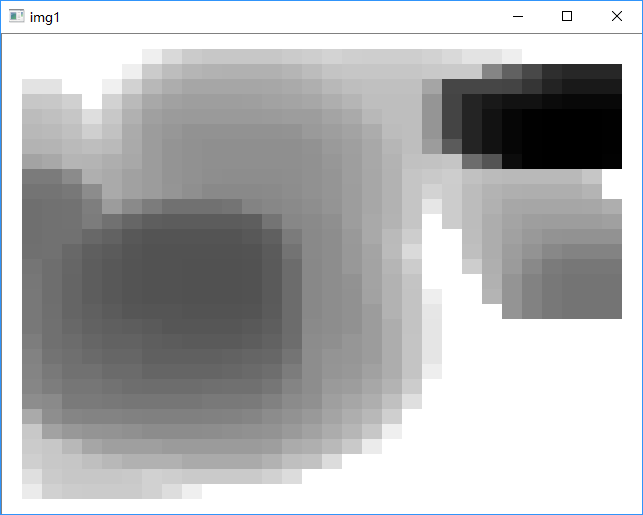1. 膨胀图像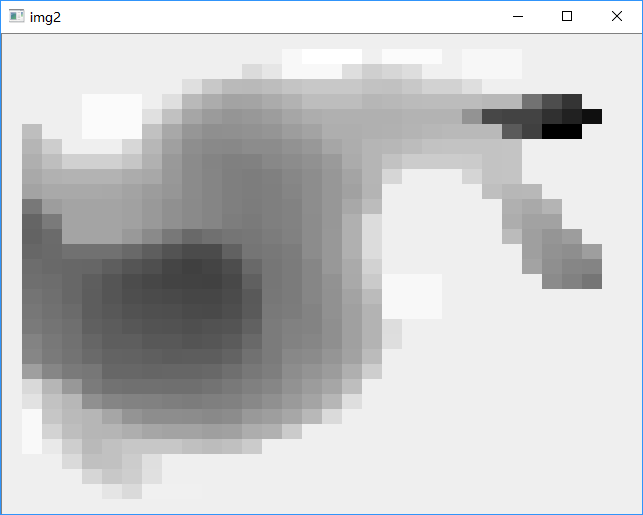## 4.总结

• 可以看出，腐蚀确实让暗像元区域变大；膨胀则让亮像元区域变大
• 还可以结合本人自己的研究方向进行下一步渐进形态学地面滤波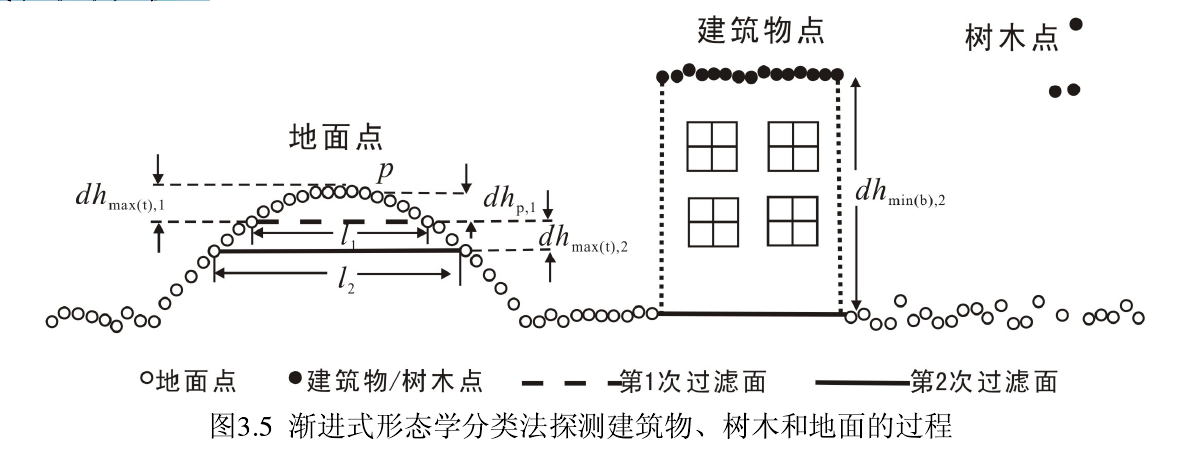• 考虑腐蚀结合膨胀，进行开闭操作

## 数字图像处理形态学运算

2018-12-20 14:51:23 qq_24163555 阅读数 183
• ###### MATLAB图像处理

介绍MATLAB提供的图像处理功能，包括图像基础知识、图像合成、空间变换、邻域和块处理、局部滤波、正交变换、数学形态学、图像分析、图像增强、图像恢复、图像分割、图像配准和图像三维重建等内容。

47课时 342分钟 216人学习 苏金明
免费试看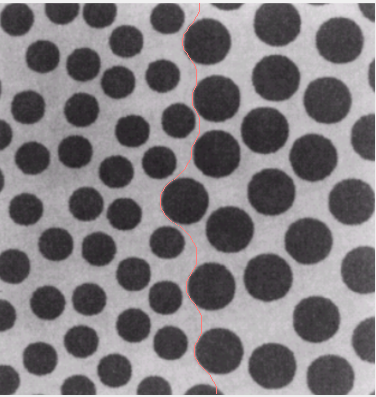clc
close all;
figure(1);imshow(I);
se=strel('ball',20,5);
I1=imclose(I,se);
figure(2),imshow(I1);
se1=strel('ball',35,5);
I2=imopen(I1,se1);
figure(3),imshow(I2);
I3=im2bw(I2,0.6);
figure(4),imshow(I3);
I4=I3;
I5=I;
[m,n]=size(I4);
for i=1:m-1
for j=1:n-1;
if(I4(i,j)==1&I4(i,j+1)==0)
I5(i,j,1)=255;
I5(i,j,2)=125;
I5(i,j,3)=122;
end
end;
end
figure(5),imshow(I5);

## 图像形态学基本运算原理

2014-05-19 08:25:22 u010545732 阅读数 3350
• ###### MATLAB图像处理

介绍MATLAB提供的图像处理功能，包括图像基础知识、图像合成、空间变换、邻域和块处理、局部滤波、正交变换、数学形态学、图像分析、图像增强、图像恢复、图像分割、图像配准和图像三维重建等内容。

47课时 342分钟 216人学习 苏金明
免费试看
﻿﻿形态学的基本运算包括腐蚀，膨胀，开运算和闭运算。

1. 腐蚀是一种消除边界点，使边界向内部收缩的过程。可以用来消除小且无意义的物体。

2. 膨胀是将与物体接触的所有背景点合并到该物体中，使边界向外部扩张的过程。可以用来填补物体中的空洞。

3. 先腐蚀后膨胀的过程称为开运算。用来消除小物体、在纤细点处分离物体、平滑较大物体的边界的同时并不明显改变其面积。

4. 先膨胀后腐蚀的过程称为闭运算。用来填充物体内细小空洞、连接邻近物体、平滑其边界的同时并不明显改变其面积。

// 腐蚀 的例子代码
//使用水平方向的结构元素进行腐蚀

IHeight，IWidth分别为图像的高度和宽度
for(j = 0; j <lHeight; j++)

for(i = 1;i <lWidth-1; i++)
{
...
//目标图像中的当前点先赋成黑色
*lpDst = (unsigned char)0;

//如果源图像中当前点自身或者左右有一个点不是黑色，
//则将目标图像中的当前点赋成白色
for (n = 0;n < 3;n++ )
{
pixel = *(lpSrc+n-1);
if (pixel == 255 )
{
*lpDst = (unsigned char)255;
break;
}

}
}

## 数字图像处理第九章——形态学图像处理

2019-05-11 08:31:12 Dujing2019 阅读数 3361
• ###### MATLAB图像处理

介绍MATLAB提供的图像处理功能，包括图像基础知识、图像合成、空间变换、邻域和块处理、局部滤波、正交变换、数学形态学、图像分析、图像增强、图像恢复、图像分割、图像配准和图像三维重建等内容。

47课时 342分钟 216人学习 苏金明
免费试看

# 数字图像处理—形态学图像处理

## （一）预备知识

1. 用数学形态学（也称图像代数）表示以形态为基础对图像进行分析的数学工具
2. 基本思想是用具有一定形态的结构元素去度量和提取图像中的对应形状以达到对图像分析识别的目的
3. 形态学图像处理的数学基础和所用语言是集合论
4. 形态学图像处理的应用可以简化图像数据， 保持它们基本的形状特性，并除去不相干的结 构
5. 形态学图像处理的基本运算有4个：膨胀、 腐蚀、开操作和闭操作

#### 1.1 集合理论中的基本概念

1. 所有像素坐标的集合均不属于集合A，记为$A^c$，由下式给出: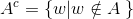这个集合称为集合A的补集

2. 集合B的反射，定义为：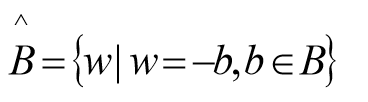即关于原集合原点对称 .

3. 集合A平移到点z=(z1,z2),表示为(A)z，定义为: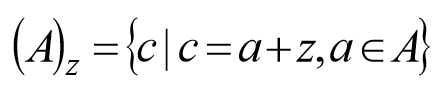#### 1.2 二值图像、集合及逻辑算子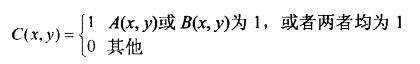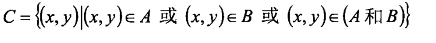1. A为图像集合，B为结构元素（集合）。
2. 数学形态学运算时B对A进行操作。
3. 结构元素要有1个原点（即结构元素参与形态学运算的参考点），可以是中心像素，原则上可选任何像素。
注意：原点可以包含在结构元素中，也可以不包含在结构元素中，但运算的结果常不相同。

f = imread('D:\数字图像处理\第九章学习\Fig0903(a).tif');
subplot(2,3,1), imshow(f);title('(a)二值图像 A:');
subplot(2,3,2), imshow(g);title('(b)二值图像 B:');
subplot(2,3,3), imshow(~f);title('(c)A的补集~A:');
subplot(2,3,4), imshow(f|g);title('(d) A和B的并集 A|B:');
subplot(2,3,5), imshow(f&g);title('(e)A和B的交集 A & B:');
subplot(2,3,6), imshow(f&~g);title('(f)A和B的差集 A&~B');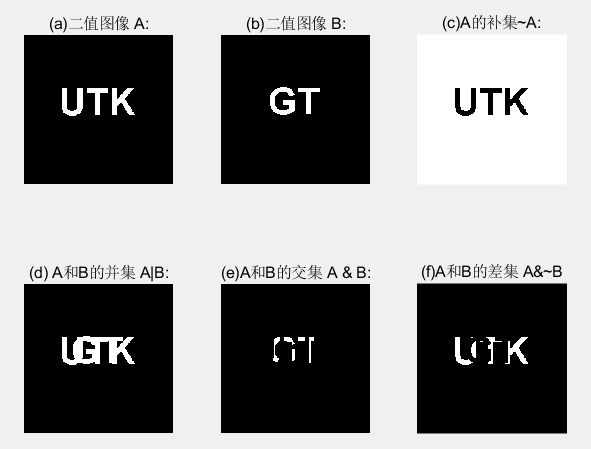## （二）膨胀和腐蚀

#### 2.1 膨胀

A和B是两个集合，A被B膨胀定义为：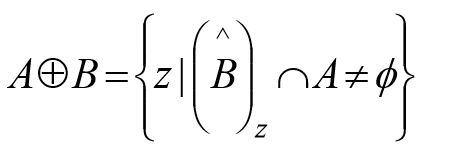1. B的反射进行平移与A的交集不为空。
2. B的反射：相对于自身原点的映象。
3. B的平移：对B的反射进行位移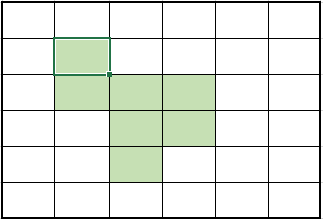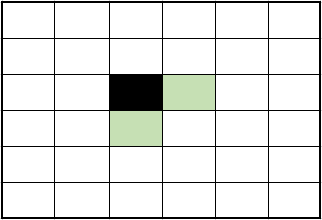(a)集合A    (b)结构元素B (黑色为原点所在)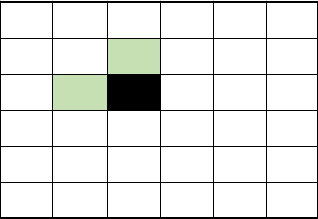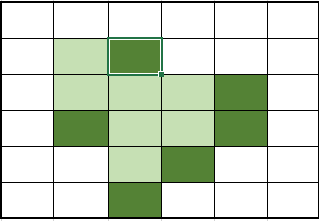(c)结构元素B的映像    (d)图中两种阴影部分（深色为扩大的部分）合起来为A+B

1. 膨胀运算只要求结构元素的原点在目标图像的内部平移，换句话说，当结构元素在目标图像上平移时，允许结构元素中的非原点像素超出目标图像的范围
2. 膨胀运算具有扩大图像和填充图像中比结果元素小的成分的作用，因此在实际应用中可以利用膨胀运算连接相邻物体和填充图像中的小孔和狭窄的缝隙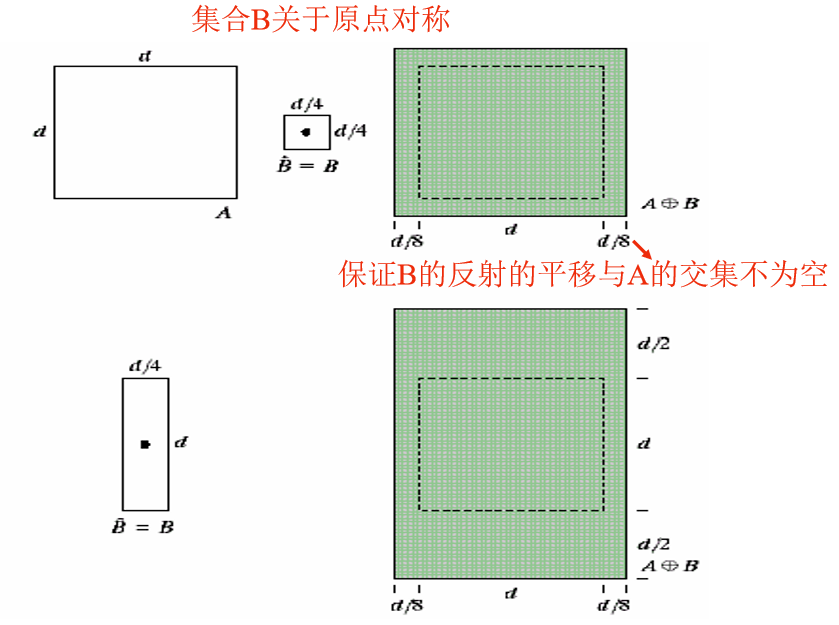D = imdilate(A,B)

A = imread('D:\数字图像处理\第九章学习\Fig0906(a).tif');
B = [0 1 0; 1 1 1; 0 1 0];   %指定结构元素由0和1组成的矩阵
A2 = imdilate(A, B);    %二值图像
subplot(1,2,1), imshow(A);title('(a)包括断开文本的输入图像:');
subplot(1,2,2), imshow(A2);title('(b)膨胀后图像:');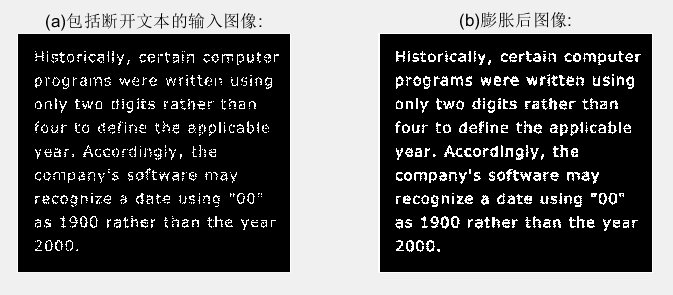#### 2.2 结构元的分解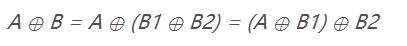B膨胀A等同于B1先膨胀A，再用B2膨胀之前的结果。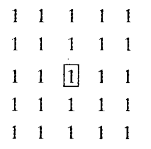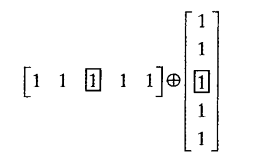#### 2.3 strel函数

se = strel(shape, parameters)

shape用于指定希望形状的字符串，parameters是描述形状信息的参数列表。

#### 2.4 腐蚀

A和B是两个集合，A被B腐蚀定义为：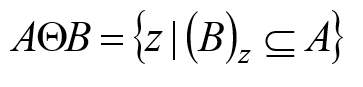1. A被 B 腐蚀是包含在A中的B由z平移的所有点z的集合。
2. B包含在A中的声明相当于B不共享A背景的任何元素。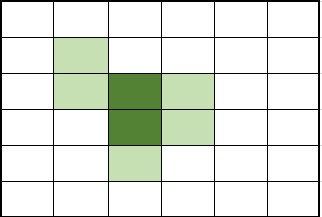(a)集合A(阴影部分)   (b)结构元素B（阴影部分，深色部分为原点）(c)阴影部分合起来为A-B

1. 当结构元素中原点位置不为1（也即原点不属于结构元素时），也要把它看作是1，也就是说，当在目标图像中找与结构元素B相同的子图像时，也要求子图像中与结构元素B的原点对应的那个位置的像素的值是1。
2. 腐蚀运算要求结构元素必须完全包括在被腐蚀图像内部：换句话说，当结构元素在目标图像上平移时，结构元素中的任何元素不能超过目标图像范围。
3. 腐蚀运算的结果不仅与结构元素的形状选取有关，而且还与原点位置的选取有关
4. 腐蚀运算具有缩小图像和消除图像中比结构元素小的成分的作用，因此在实际应用中，可以利用腐蚀运算去除物体之间的粘连，消除图像中的小颗粒噪声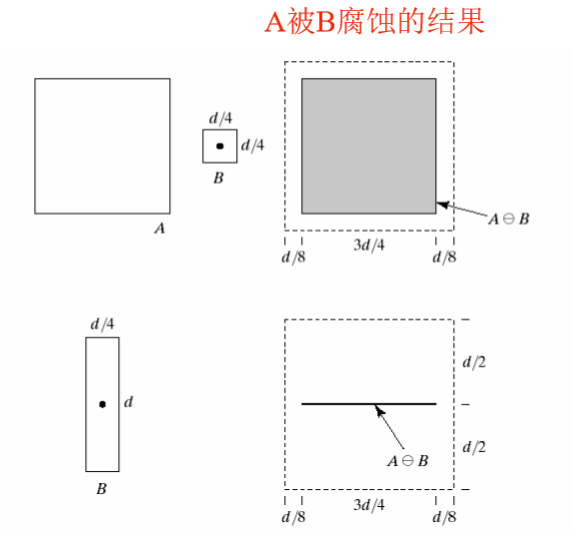A2 = imerode(A, se)

f = imread('D:\数字图像处理\第九章学习\Fig0908(a).tif');
se = strel('disk', 10);
g = imerode(f, se);
se = strel('disk', 5);
g1 = imerode(f, se);
g2 = imerode(f, strel('disk', 20));
subplot(2,2,1), imshow(f);title('(a)原始图像的尺寸为480x480像素:');
subplot(2,2,2), imshow(g);title('(b)用半径为10的圆形腐蚀:');
subplot(2,2,3), imshow(g1);title('(c)用半径为5的圆形腐蚀:');
subplot(2,2,4), imshow(g2);title('(d)用半径为20的圆形腐蚀');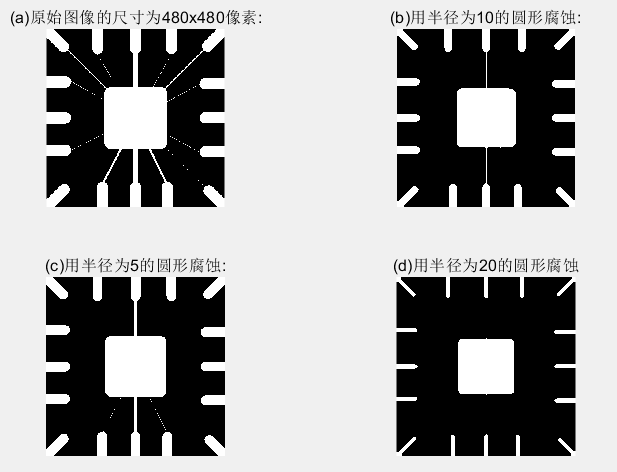## （三） 膨胀与腐蚀的结合

#### 3.1 开操作和闭操作

1. 使图像的轮廓变得光滑，断开狭窄的间断和消除细的突出物。
2. 使用结构元素B对集合A进行开操作，定义为：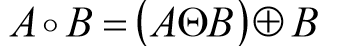先用B对A腐蚀，然后用B对结果膨胀。
3. 与开操作等价的数学表达式为: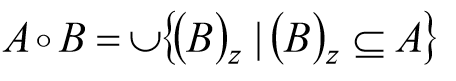4. A o B 的边界通过B中的点完成。
5. B在A的边界内转动时，B中的点所能到达的A的边界的最远点。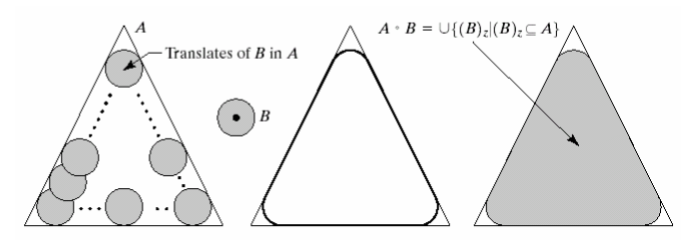6. A o B 是 A的子集合。
7. 如果C是D的子集，则 C o B是 D o B的子集。
8. (A o B) o B = A o B

1. 同样使图像的轮廓变得光滑，但与开操作相反，它能消除狭窄的间断和长细的鸿沟，消除小的孔洞，并填补轮廓线中的裂痕。
2. 使用结构元素B对集合A进行闭操作，定 义为：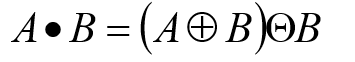先用B对A膨胀，然后用B对结果腐蚀。
3. A . B的边界通过B中的点完成 。
4. B在A的边界外部转动 :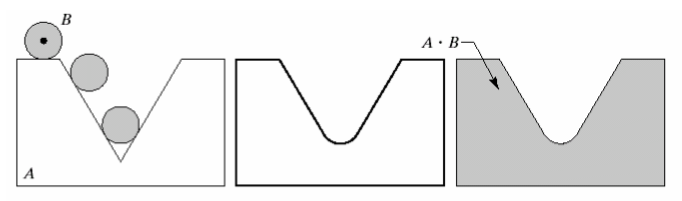5. A 是 A . B的子集合。
6. 如果C 是 D 的子集 ， 则C . B 是 D . B的子集。
7. (A . B) . B = A . B

C = imopen(A, B)

C = imclose(A, B)

A为二值图像，B为0，1矩阵组成，并且是指定结构元素。

f = imread('D:\数字图像处理\第九章学习\Fig0910(a).tif');
se = strel('square', 40);
fo = imopen(f, se);
fc = imclose(f, se);
foc = imclose(fo, se);
subplot(2,2,1), imshow(f), title('(a)原图');
subplot(2,2,2), imshow(fo), title('(b)开操作');
subplot(2,2,3), imshow(fc), title('(c)闭操作');
subplot(2,2,4), imshow(foc), title('(d) (b)的闭操作结果');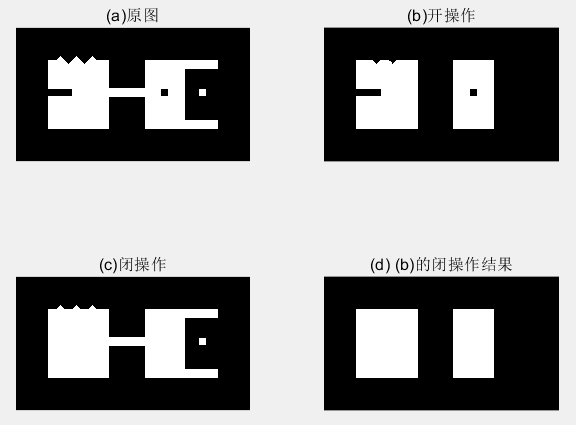1. 图(a)中的图像设计了一些用于演示开操作和闭操作的特征，比如细小突起、细的桥接点、几个弯口、孤立的小洞、 小的孤立物和齿状边缘。
2. 图 (b)显示了结果。注意，从图中可以看出，细的突出和外部点的边缘的不规则部分被去除掉了，细的桥接和小的孤立物也被去除了。
4. 图 (d)显示了平滑过的物体。

f = imread('D:\数字图像处理\第九章学习\Fig0911(a).tif');
se = strel('square', 6);
fo = imopen(f, se);
foc = imclose(fo, se);
subplot(1,3,1), imshow(f), title('(a)带噪声的指纹图像');
subplot(1,3,2), imshow(fo), title('(b)图像的开操作');
subplot(1,3,3), imshow(foc), title('(c)先用开操作，再用闭操作');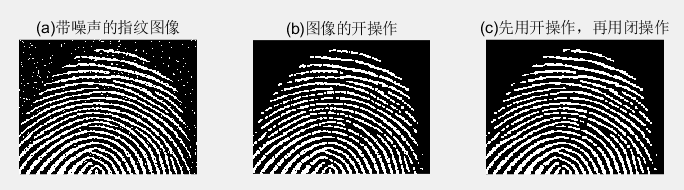1. 图(a)是受噪声污染的指纹二值图像，噪声为黑色背景上的亮元素和亮指纹部分的暗元素。
2. 图(b)所示的图像。发现，对图像进行开操作可以去除噪声点，但是这种处理在指纹的纹脊上又引入一些缺口
3. 图( c )显示了最终结果。在这个结果中，大多数噪声被消除了，开运算的闭运算可以给指纹填充缺口，但是指纹纹路并没有完全恢复 。

#### 3.2 击中或击不中变换

A中对B进行的匹配（击中）表示为：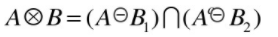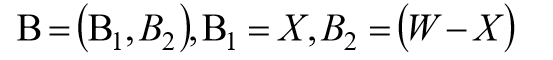B1是由与一个对象相联系的B元素构成的集合，B1是由与一个对象相联系的B元素构成的集合。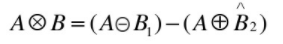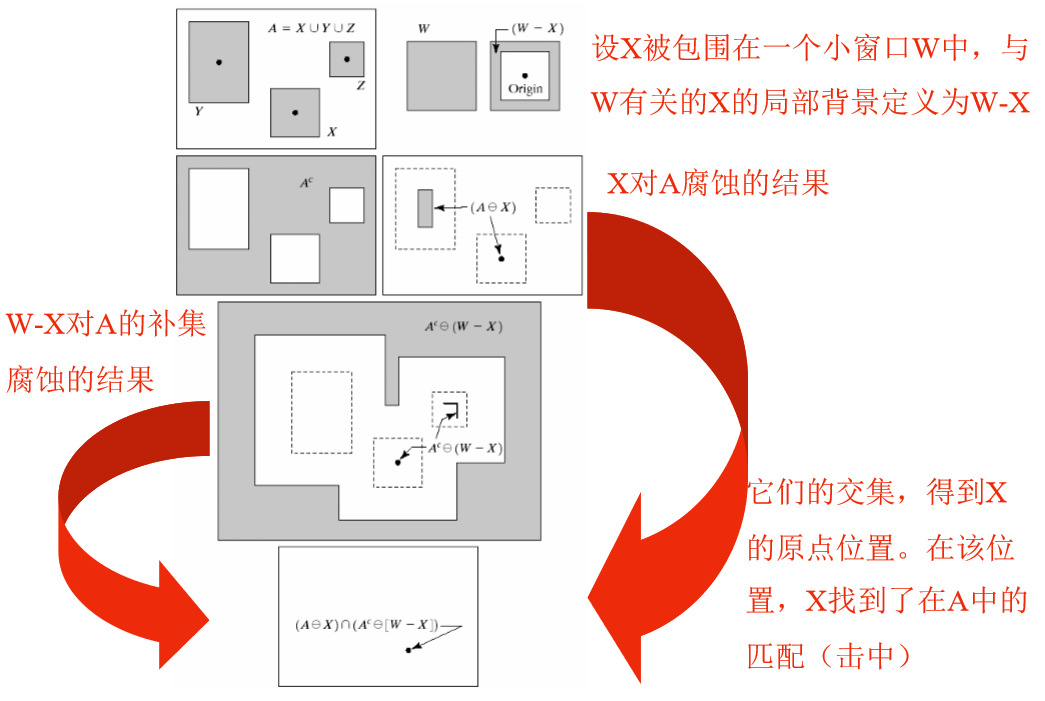C = bwhitmiss(A, B1, B2)

f = imread('D:\数字图像处理\第九章学习\Fig0913(a).tif');
B1 = strel([0 0 0;0 1 1; 0 1 0]);
B2 = strel([1 1 1;1 0 0;1 0 0]);
g = bwhitmiss(f,B1,B2);
subplot(1,2,1), imshow(f), title('(a)原始图像');
subplot(1,2,2), imshow(g), title('(b)击中、击不中变换的结果');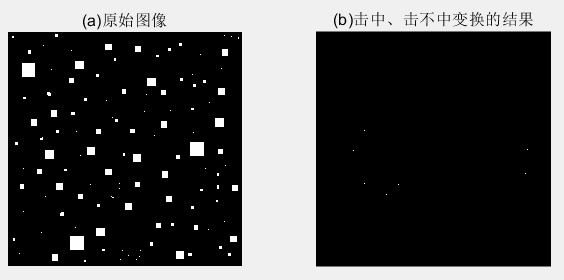1. 图(a)显示了包括各种尺寸的正方形图像。我们要定位有东、南相邻像素(这些 “击中”）和没有东北、北、西北、西和西南相邻像素(这些 “击不中”）的前景像素。这些要求导致以下B1,B2两个结构元。这两个结构元都不包括东南邻域像素，这称为不关心像素。用函数 bwhitmiss 来计算变换。
2. 图 (b)中的每个单像素点都是图 (a)中物体左上角的像素。图 (b)中是放大后的像素，以便更清晰。bwhitmiss的替代语法可以把Bl 和 B2 组合成间隔矩阵。只要 B1等于 1 或-1，B2 等于 1, 间隔矩阵就等于 1。对于不关心像素，间隔矩阵等于 0。

#### 3.3 bwmorph函数

g = bwmorph(f, operation, n);

f 是输入的二值图像，operation 是指定所希望运算的字符串，n 是指定重复次数的正整数。

f = imread('D:\数字图像处理\第九章学习\Fig0911(a).tif');
g1 = bwmorph(f, 'thin',1);
g2 = bwmorph(f, 'thin',2);
ginf = bwmorph(f,'thin', Inf);
subplot(1,4,1),imshow(f);title('(a)指纹图像:');
subplot(1,4,2),imshow(g1);title('(b)细化一次后的指纹图像:');
subplot(1,4,3),imshow(g2);title('(c)细化两次后的图像:');
subplot(1,4,4),imshow(ginf);title('(d)一直细化到稳定状态的图像：');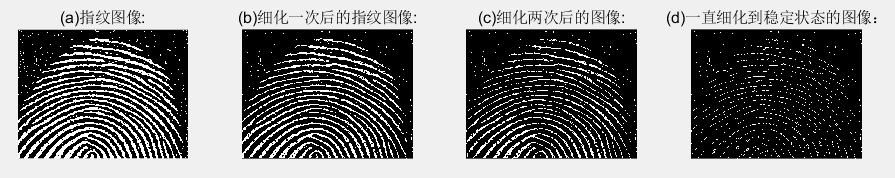f = imread('D:\数字图像处理\第九章学习\Fig0916(a).tif');
fs = bwmorph(f,'skel',Inf);
for k = 1:5
fa = fs & ~endpoints(fs);
end
subplot(1,3,1),imshow(f);title('(a)骨头图像:');
subplot(1,3,2),imshow(fs);title('(b)使用函数 bwmorph 得到的骨豁:');
subplot(1,3,3),imshow(fa);title('（c)使用函数 endpoint 裁剪后的骨豁：');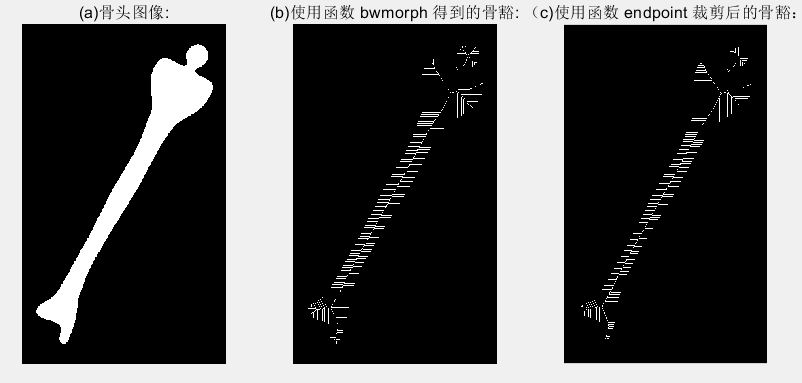## （四）标记连通分量

[L, num] = bwlabel (f, conn)

f 是输入二值图像，coon指定希望的连接方式（不是4连接就是8连接），输出L叫做标记矩阵，函数num则给出找到的连通分量总数。

f = imread('D:\数字图像处理\第九章学习\Fig0917(a).tif');
imshow(f);title('(a)标注连通分量原始图像:');
[L,n]=bwlabel(f);        %L为标记矩阵，n为找到连接分量的总数
[r,c]=find(L==3);        %返回第3个对象所有像素的行索引和列索引
rbar=mean(r);
cbar=mean(c);
figure,imshow(f);title('(b)标记所有对象质心后的图像:');
hold on            %保持当前图像使其不被刷新
for k=1:n
[r,c]=find(L==k);
rbar=mean(r);
cbar=mean(c);
plot(cbar,rbar,'Marker','o','MarkerEdgeColor','k',...
'MarkerFaceColor','k','MarkerSize',10);
plot(cbar,rbar,'Marker','*','MarkerFaceColor','w'); %其中的marker为标记
end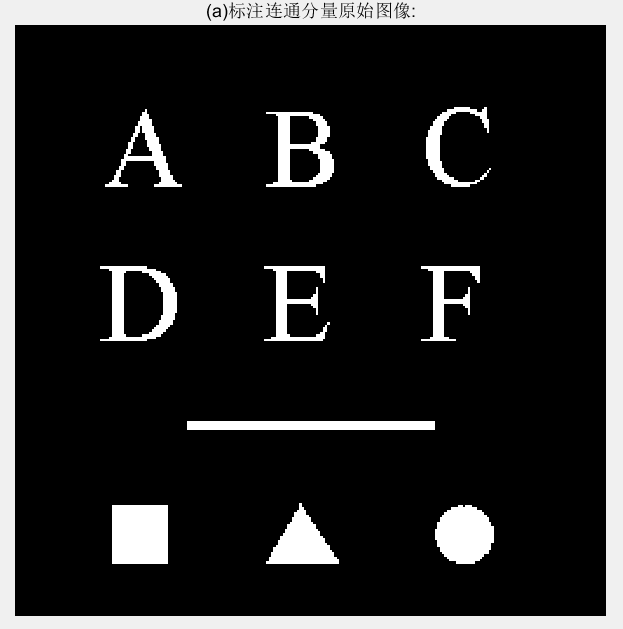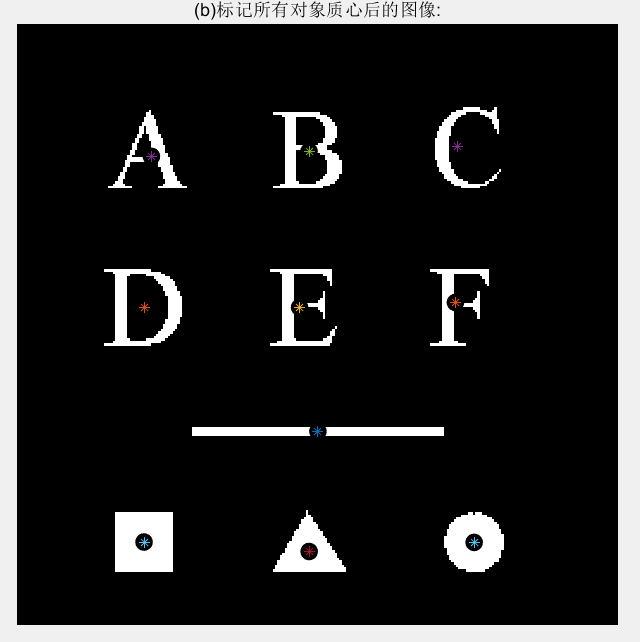## （五）形态学重建

1. 将h1初始化为标记图像f。
2. 创建结构元素 ：B = ones(3)。
3. 重复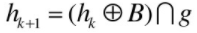直到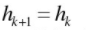其中，标记f必须是g的一个子集。

#### 5.1 通过重建进行开操作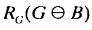f = imread('D:\数字图像处理\第九章学习\Fig0917(a).tif');
subplot(3,2,1),imshow(f);title('(a)重构原始图像');
fe=imerode(f,ones(51,1));%竖线腐蚀
subplot(3,2,2),imshow(fe);title('(b)使用竖线腐蚀后的结果');
fo=imopen(f,ones(51,1));%竖线做开运算
subplot(3,2,3),imshow(fo);title('(c)使用竖线做开运算结果');
fobr=imreconstruct(fe,f);%fe做标记
subplot(3,2,4),imshow(fobr);title('(d)使用竖线做重构开运算');
ff=imfill(f,'holes');%对f进行孔洞填充
subplot(3,2,5),imshow(ff);title('(e)对f填充孔洞后的图像');
fc=imclearborder(f,8);%清除边界，2维8邻接
subplot(3,2,6),imshow(fc);title('(f)对f清除边界后的图像');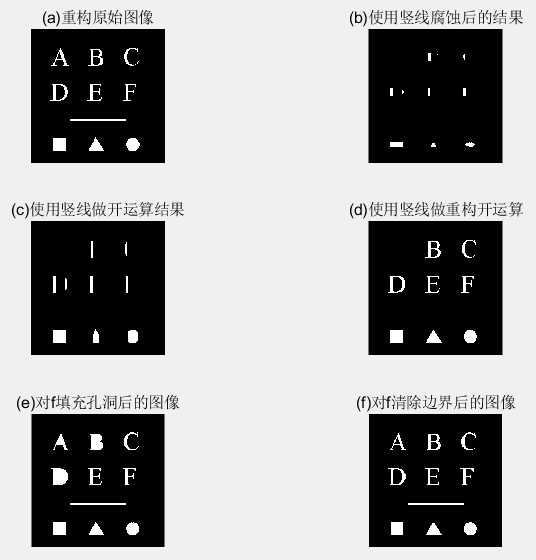1. 传统开运算中，腐蚀去除掉小对象，随后的膨胀恢复原始对象形状，但受元素结构影响，恢复的往往不是很精确。
2. 重构则能精确恢复原始图像。

#### 5.2 填充孔洞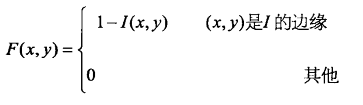g = imfill(f,‘holes’);

#### 5.3 清除边界物体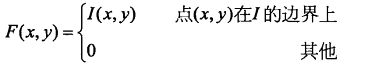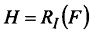g = imclearborder(f,conn)

f 是输入图像，g 是结果。conn 的值不是 4 就是 8(默认)。 物体更亮且与图像边界相连接的结构。

## （六）灰度级形态学

#### 6.1 膨胀和腐蚀

1. 使用结构元素b对f的灰度膨胀定义为：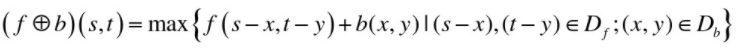其中，$D_f$$D_b$分别是f和b的定义域，f和b是函数而不是二值形态学情况中的集合。

2. 当结构元素b是平坦的，即b(x,y)在其定义域内都为0时：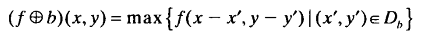1. 使用结构元素b对f的灰度腐蚀定义为：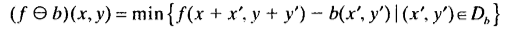其中，$D_f$$D_b$分别是f和b的定义域。

2. 当结构元素b是平坦的，即b(x,y)在其定义域内都为0时：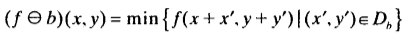f = imread('D:\数字图像处理\第九章学习\Fig0923(a).tif');
se=strel('square',3);  %构造了一个平坦的3x3的结构元素
gd=imdilate(f,se);    %对原图像进行膨胀操作
ge=imerode(f,se);     %对原图像进行腐蚀操作
subplot(3,2,1);imshow(f,[]);title('(a)原始图像');
subplot(3,2,2),imshow(gd,[]);title('(b)膨胀的图像');
subplot(3,2,3),imshow(ge,[]);title('(c)腐蚀的图像');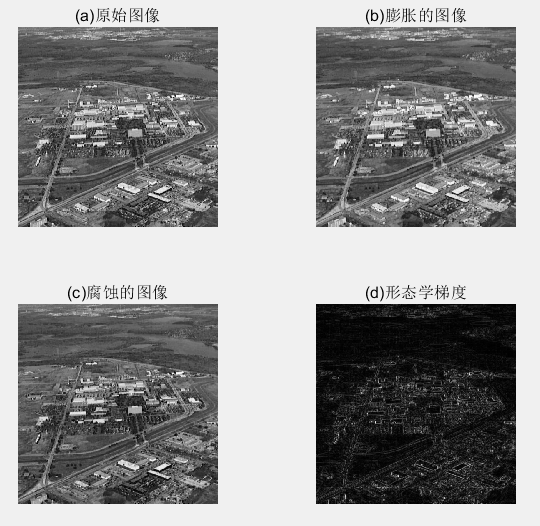1. 膨胀得到的图像比原图像更明亮，并且减弱或消除小的，暗的细节部分。即比原图像模糊。
2. 腐蚀得到的图像更暗，并且尺寸小，明亮的部分被削弱 。

#### 6.2 开操作和闭操作

1. 在灰度图像中，开操作的表达式与二值图像拥有相同的形式。2. 把一幅图像看做是一个三维表明，其亮度值代表xy平面上的高度值，则当结构元素b在f下面活动时，结构元素的任何部分的最高值构成了开运算的结果。
3. 先进行腐蚀操作可以除去小的亮的图像细节，但这样会使图像变暗，接下来进行膨胀操作增强图像的整体亮度。

1. 在灰度图像中，闭操作的表达式与二值图像拥有相同的形式。2. 当结构元素b在f的上面活动时，结构元素的任何部分的最低值构成了闭运算的结果 。
3. 先通过膨胀操作除去图像中的暗细节，同时增加图像的亮度，接下来对图像进行腐蚀，而不会将膨胀操作除去的部分重新引入图像中。

f = imread('D:\数字图像处理\第九章学习\Fig0925(a).tif');
subplot(3,2,1),imshow(f);
title('(a)木钉图像原图');
se=strel('disk',5);     %disk其实就是一个八边形
fo=imopen(f,se);        %经过开运算
subplot(3,2,2),imshow(f);
title('(b)使用半径5的disk开运算后的图像');
foc=imclose(fo,se);
subplot(3,2,3),imshow(foc);
title('(c)先开后闭的图像');
focd=imclose(f,se);
subplot(3,2,4),imshow(focd);
title('(d)原始图像的闭操作');
foce=imopen(focd,se);
subplot(3,2,5),imshow(foce);
title('(e)先闭后开的图像');
fasf=f;
for i=2:5
se=strel('disk',i);
fasf=imclose(imopen(fasf,se),se);
end
subplot(3,2,6),imshow(fasf);
title('(f)使用开闭交替滤波后图像');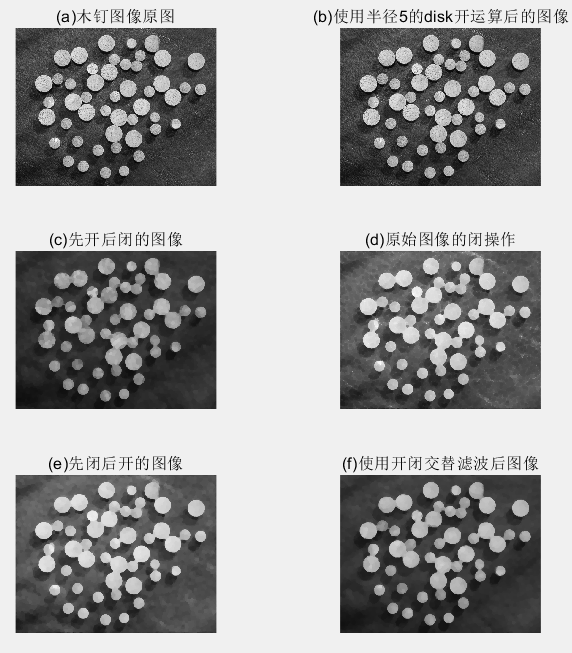1. 图 (b)显示了开操作的图像 fo, 在这里，我们看到，亮区域己经被调低了(平滑)，木钉上的暗条文几乎没有受影响。
2. 图 (c )显示了开操作的闭操作 foe。现在我们注意到，暗区域已经被平滑得很好了，结果是整个图像得到全部平滑。这种过程通常叫做开-闭滤波。先开运算后闭运算构成噪声滤波器，用来平滑图像并去除噪声。
3. 图 (d)显示了原始图像的闭操作结果。木钉上的暗条文已经被平滑掉了，主要留下了亮的细节(注意背景中的亮条文)。
4. 图 (e)显示了这些条文的平滑和木钉表面的进一步平滑效果。最终结果是原始图像得到全部平滑。

f = imread('D:\数字图像处理\第九章学习\Fig0926(a).tif');
g = f>=(255*graythresh(f));
se=strel('disk',100);
fo=imopen(f,se);
f2=imsubtract(f,fo);
g1 = f2>=(255*graythresh(f2));
subplot(2,3,1),imshow(f);
title('(a)原始图像');
subplot(2,3,2),imshow(g);
title('(b)经过阈值处理后的图像');
subplot(2,3,3),imshow(f);
title('(c)原图开运算后的图像');
subplot(2,3,4),imshow(f2);
title('(d)原图减去开运算');
subplot(2,3,5),imshow(g1);
title('(e)最终结果');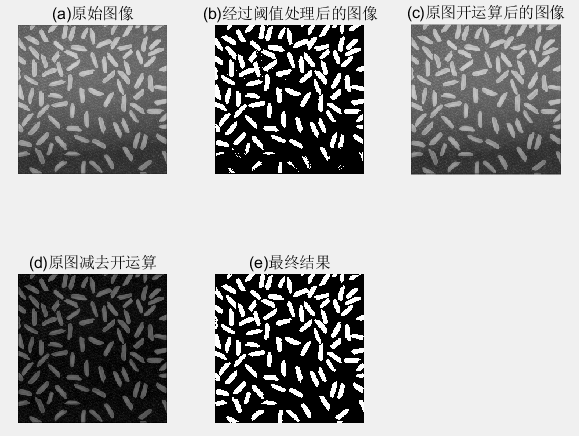1. 图 (a) :显示了一幅米粒的图像f，图像下部的背景比上部的黑。这样的话，对不平坦的亮度进行阈值处理会很困难。
2. 图 (b) "是阈值处理方案，图像顶端的米粒被很好地从背景中分离开来，但是图像底部的米粒没有从背景中正确地提取出来。
3. 图(c ):对图像进行开操作，可以产生对整个图像背景的合理估计。
4. 图(d) :把图(c )从原始图像中减去，生成一幅拥有合适的均勾背景的米粒图像.
5. 图(e):显示了新的经阈值处理后的图像。注意，改进效果超过了图 (b)。

f = imread('D:\数字图像处理\第九章学习\Fig0926(a).tif');
sumpixels=zeros(1,36);
for k=0:35
se=strel('disk',k);
fo=imopen(f,se);
sumpixels(k+1)=sum(fo(:));
end
%可以看到，连续开运算之间的表面积会减少
plot(0:35,sumpixels),xlabel('k'),ylabel('surface area');
title('(a)表面积和结构元素半径之间的关系');
figure,plot(-diff(sumpixels));%diff()函数为差分或者近似倒数，即相邻2个之间的差值
xlabel('k'),ylabel('surface area reduction');
title('(b)减少的表面积和结构元素半径之间的关系');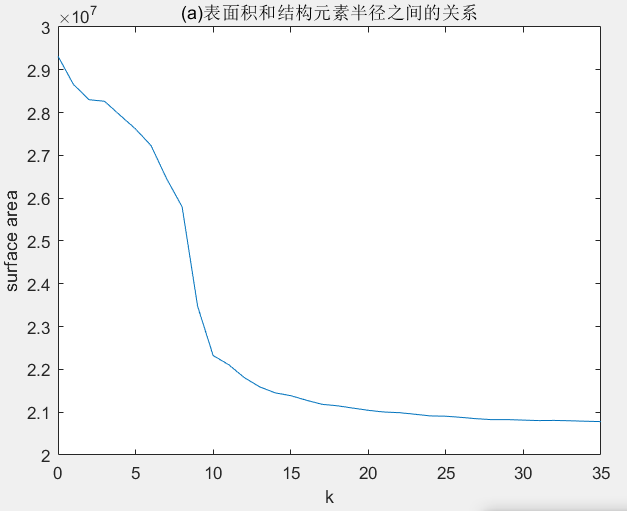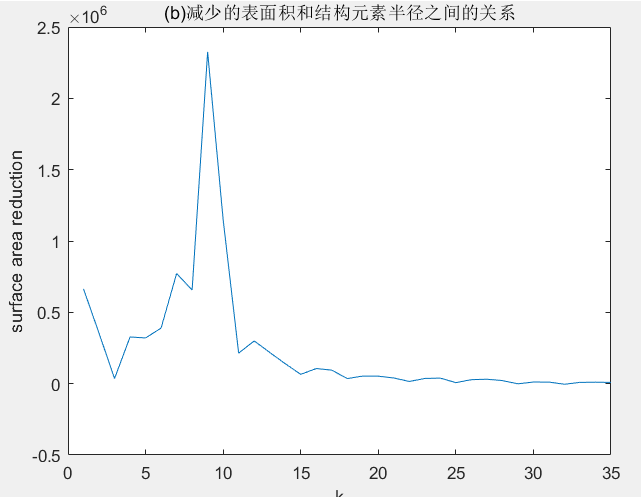1. (a)连续开运算之间的表面积会减小。
2. (b)图峰值表明出现了大量的有着这种半径的对象。

#### 6.3 重建

1. h极小值变换：标记图像是由掩膜挑选ing减去常量所得。
2. 开运算重建：先腐蚀后重建。
3. 闭运算重建：对图像求补、计算其开操作重建并对结果求补。

f = imread('D:\数字图像处理\第九章学习\Fig0930(a).tif');
subplot(3,3,1),imshow(f);
title('(a)原图像');
f_obr=imreconstruct(imerode(f,ones(1,71)),f);
subplot(3,3,2),imshow(f_obr);
title('(b)重建的开操作');
f_o=imopen(f,ones(1,71));
subplot(3,3,3),imshow(f_o);
title('(c)开操作');
f_thr=imsubtract(f,f_obr);    %顶帽重构
subplot(3,3,4),imshow(f_thr);
title('(d)重建的顶帽操作');
f_th=imsubtract(f,f_o)    %标准顶帽运算，方便比较
subplot(3,3,5),imshow(f_th);
title('(e)顶帽操作');
g_obr=imreconstruct(imerode(f_thr,ones(1,11)),f_thr);
subplot(3,3,6),imshow(g_obr);
title('(f)用水平线对(b)经开运算后重建图');
g_obrd=imdilate(g_obr,ones(1,2));
subplot(3,3,7),imshow(g_obrd);
title('(g)使用水平线对(f)进行膨胀');
f2=imreconstruct(min(g_obrd,f_thr),f_thr);
subplot(3,3,8),imshow(f2);
title('(h)最后的重建结果');